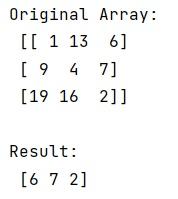# Access the ith column of a NumPy multidimensional array

In this tutorial, we will learn how to access the ith column of a NumPy multidimensional array? By Pranit Sharma Last updated : May 23, 2023

Suppose that we are given a multidimensional array, and we need to find a way to access the ith column of this multidimensional array.

## How to access the ith column of a NumPy multidimensional array?

To access the ith column of a NumPy multidimensional array, we will transpose the given array using the T property and pass the index as a slicing index. The transpose of an array is created by using the first row of the array as the first column of the new array, the second row of the array as the second column of the new array, and so on.

Let us understand with the help of an example,

## Python program to access the ith column of a NumPy multidimensional array

```# Import numpy
import numpy as np

import sys

# Creating a numpy array
arr = np.array([[1, 13, 6], [9, 4, 7], [19, 16, 2]])

# Display Original Array
print("Original Array:\n",arr,"\n")

# Using Transpose property (T)
res = arr.T

# Display Result
print("Result:\n",res,"\n")
```

### Output